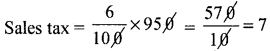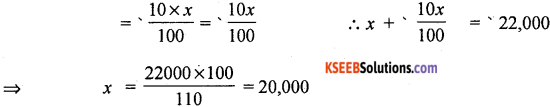# 1st PUC Basic Maths Question Bank Chapter 11 Percentages of Profit and Loss Sales tax, Vat

Students can Download Basic Maths Chapter 11 Percentages of Profit and Loss Sales tax, Vat Questions and Answers, Notes Pdf, 1st PUC Basic Maths Question Bank with Answers helps you to revise the complete Karnataka State Board Syllabus and score more marks in your examinations.

## Karnataka 1st PUC Basic Maths Question Bank Chapter 11 Percentages of Profit and Loss Sales tax, Vat

Question 1.
Ravi purchased a pair of shoes costing ‘950. Calculate the total amount to be paid by him, if the rate of sales tax is 7%.
Sales price = Rs. 950Total amount paid by Ravi = ‘950 + ’57 = ‘1007.

Question 2.
Sneha purchased confectionery goods costing 165 on which the rate of sales tax is 6% and some tooth paste, cold cream, soap etc. costing ‘ 550 which the rate of sales tax is 10%. If she gives 1000 note to the shop keeper, What money will be return to Mrs Sneha?
Price of confectionary goods with sales tax = ’65 + 6% + 165 = ‘174.90
Price of toothpaste, cold cream soap with tax = ‘550 + 10% of 550 = 550 + 55 = 605
∴ the average to be paid = 174.90 + 605 = 774.90
Amount to be returned by shop keeper = 1000 – 774.90 = ‘225.90 paise.Question 3.
The price of an article inclusive of sales tax of 15% is 3450. Find its marked price if the sales tax is reduced to 6%. How much less does the customer pay for the article?
Let the marked price = ‘x
∴ x + 15% of x = 3450
⇒ $$\frac{115 x}{100}$$ = 3050 ⇒ x = 3000
∴ Market price of the article = ‘3000
Since new sales tax = 60%
Now the customer will pay = ‘3000 + 6% of ‘3000 = $$\frac{106}{100}$$ × ‘3000 = 3180
∴ customer will pay for the article = 3450 – 3180 = 270 less

Question 1.
A shop keeper buys an article for Rs. 1500 and spends20% of the cost on its packing transportation etc. Then he marked price on it. If he sells the article for ‘2,452.50 including 9% sales tax on the price marked. Find its profit as percent.
Let marked price of the article be ‘x.
Sales tax price = 9% of x = $$\frac{9 x}{100}$$
According to given statement n + $$\frac{9 x}{100}$$ = 2452.50
⇒ $$\frac{100 x+9 x}{100}$$ = 2452.50 ⇒ x = 2452.50 × $$\frac{100}{109}$$ = 2,250
∴ Marked price = ‘2,250 = selling price
Since the shopkeeper buys for ‘1500 and spends 20% of the cost as overhead.
∴ Total cost price of the article = ‘1500 + 20% ‘1500 = ‘1500 + ‘300= ‘1800
Profit = Selling price – total cost price = 2250 – 1800 = 450
∴ Profit % = $$\frac{450}{1800}$$ × 100 = 22%Question 2.
Prabha bought an article ‘374. Which included a discount of 15% on the market price and a sales tax of 10% on the reduced price. Find the market price of the article.
Let the market price of the article be ‘x
∴ Discount 15% of x = $$\frac{15}{100} \times x=\frac{3 x}{20}$$
⇒ Remaining cost = x – $$\frac{3 x}{20}=\frac{17 x}{20}$$
Sales tax = 10%
∴ price paid by prabha = $$\frac{110}{100} \times \frac{17 x}{20}=\frac{187 x}{200}$$
Given $$\frac{187 x}{200}$$ = 374 = x = 374 = $$\frac{200}{187}$$ = 400
∴ Market price = ‘400

Question 3.
A Shopkeeper buys an article at a rebate of 20% on the printed price. He spends ” 40 on transportation of article. After charging a sales tax of 7% on the printed price he sells the article for ‘1,070. Find his gain on percent.
Let printed price of the article be ‘ x
⇒ Sales tax on it = 7% of ‘x = $$\frac{7 x}{100}$$
By data x$$\frac{7 x}{100}$$ = 1070 ⇒ x = 1000
∴ Printed price = ‘1000
Again the shop keeper buy the article at 20% rebate.
∴ The cost price to the shop keeper = ‘1000 – 20% of 1000 = ‘ 800
Since he spends ’40 on the transportation of the article
⇒ Total cost price = ‘800 + ’40 = ‘840
The selling price = printed price = ‘1000
⇒ Profit = ‘100 – ‘840 =’160
And profit = $$\frac{160}{840}$$ × 100 = 19 $$\frac{1}{21} \%$$Question 1.
A Shopkeeper sales an article at its marked price ‘7,500 and charges sales tax at the rate of 12% from the customer. If the shopkeeper pays a VAT of ‘180, calculate the price (inclusive of tax) paid by the shopkeeper.
Since the shopkeeper sells the article for ‘7,500 and changes sales tax at the rate of 12%
∴ tax charged by the shopkeeper = 12% of ‘7500
= $$\frac{12}{100}$$ x 7500 = 900
VAT = Tax charge – tax paid ,
⇒ ‘180 = ‘900 – Taxpaid
Tax paid by the shopkeeper = ‘ 900 – ‘180 =’ 720
If the shopkeeper buys the article for ‘ x,
Tax on it = 12% of ‘x = ‘720
⇒ x = ‘6,000 ∴ The price (inclusive of tax)
paid by the shopkeeper = ‘6000 + ‘720 = ‘6720.

Question 2.
During the financial year a shopkeeper purchased goods worth ‘4,15,000 and paid a total tax of ‘38,000. His sales during the period consisted of a taxable turnover of ‘50,000 for goods taxable at 5% and ‘3,20,000 for goods takable at 12%. He also sold tax exempted goods worth ‘ 45,000. Calculate his tax liability for the financial year.
Goods taxable at 5% turover = ‘ 50,000
Tax = 50% of ‘50,000 = ‘ 2,500
of goods taxable at 125, turover = ‘3,20,000
tax = 12% of ‘3,20,000 = ‘38,400
Given tax exampled sales = ‘45,000
Total tax = ‘2,500 + ‘38,400 = ‘40,900
Tax paid = ‘38,000
‘ Net tax payable = Total tax changed – tax paid
= ‘40,900 – ‘38,000
=’2,900
∴ Tax liability (under VAT) = ‘2,900Question 3.
A manufacturing company sold a commodity to its distributor for ‘22,000 including VAT. The distributor sold the commodity to a retailer for ‘ 22,000 excluding tax and the retailer sold it to the customer for ‘ 25,000 plan tax (under VAT). If the rate of tax is 10%. What was the:
(i) Sale price of the commodity for the manufacture?
(ii) An amount of tax received by the state government on the sale of the commodity?
(i) Let the price of the commodity for the manufacture be ‘x, tax is 10%.
∴ tax charged by the manufactures = 10% of C.P∴ The sale price of the commodity for the manufacture = 20,000

(ii) VAT collected by the manufacture = tax charged by the manufacturesince the distributor has sold the commodity tot he retailer for ‘22,000
∴ tax collected by the distributor = 10% of22,000
= $$\frac{10}{100}$$ × 22000 = ‘2200 = paid by retailer
VAT to be deposited by the distributor = ‘2200 – ‘2000 = ‘200
Tax collected by retailer = 10% of 25000
= $$\frac{10}{100}$$ × 25,000 = ‘2500
∴ VAT to be paid by the retailer = ‘2500 – ‘2200 = ‘300
∴ Amount of the (under VAT) received by the state government
= ‘2000 + ‘200 + ‘300 = ‘2500

error: Content is protected !!